Refer to our Texas Go Math Grade 7 Answer Key Pdf to score good marks in the exams. Test yourself by practicing the problems from Texas Go Math Grade 7 Unit 3 Study Guide Review Answer Key.

Module 5 Experimental Probability

Find the probability of each event. (Lesson 5.1)

Question 1.
Rolling a 5 on a fair number cube.
Total number of cube: Possibilities 1, 2, 3, 4, 5, 6, so 6.
Number: A regular cube has only one side with number five, so 1 possibility.
P (rolling a 5) =The probability of rolling a 5 is $$\frac{1}{6}$$.

Question 2.
Picking a 7 from a standard deck of 52 cards. A standard deck includes 4 cards of each number from 2 to 10.
There are 4 cards with 7, so number of cards with 7 is 4.
P (picking a 7) =The probability to pick a number 7 is $$\frac{1}{13}$$.

Question 3.
Picking a blue marble from a bag of 4 red marbles, 6 blue marbles, and 1 white marble.
Total number of marbles in all color: 4 + 6 + 1 = 11
P (picking a blue marble) =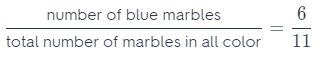The probability of picking a blue marble is $$\frac{6}{11}$$.

Question 4.
Rolling a number greater than 7 on a 12-sided number cube.
Number of sides with number greater than 7 is side with 8, 9, 10, 11, 12, so 5 possibilities for number greater than 7.
P(greater than 7) =The probability of rolling a number greater than 7 is $$\frac{5}{12}$$.

Question 5.
Christopher picked coins randomly from his piggy Penny Nickel Dime Quarter bank and got the numbers of coins shown in the table. Find each experimental probability. (Lessons 5.2, 5.3)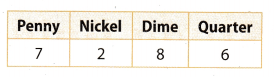Total number of possible outcomes is:
7 + 2 + 8 + 6 = 23

a. The next coin that Christopher picks is a quarter. __________________
P (picking a quarter) =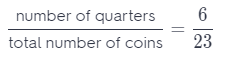The probabiLity of picking a quarter is $$\frac{6}{23}$$

b. The next coin that Christopher picks is not a quarter. _______________
P (not picking a quarter) = 1 – P (picking a quarter) = 1 – $$\frac{6}{23}$$ = $$\frac{23}{23}$$ – $$\frac{6}{23}$$ = $$\frac{17}{23}$$
The probability of not picking a quarter is $$\frac{17}{23}$$

c. The next coin that Christopher picks is a penny or a nickel. __________
P (picking a penny or nickel) =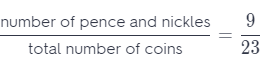The probability of picking a penny or nickel is $$\frac{9}{23}$$

Question 6.
A grocery store manager found that 54% of customers usually bring their own bags. In one afternoon, 82 out of 124 customers brought their own grocery bags. Did a greater or lesser number of people than usual bring their own bags? (Lesson 5.4)
Use proportion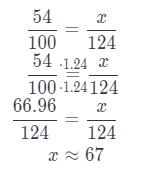The number of people that usually bring their own bags is greater that afternoon because 82 > 67

Module 6 Theoretical Probability

Find the probability of each event. (Lessons 6.1, 6.2)

Question 1.
Graciela picks a white mouse at random from a bin of 8 white mice, 2 gray mice, and 2 brown mice.
Number of possible ways is 8 because we have 8 white mices.
Total number of possible outcomes is 12 because we have in total 12 mices in white, grey and brown color.
P (picking a white mouse) =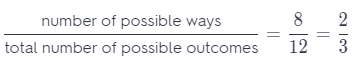The probability of picking a white mouse is $$\frac{2}{3}$$

Question 2.
Theo spins a spinner that has 12 equal sections marked 1 through 12. It does not land on 1.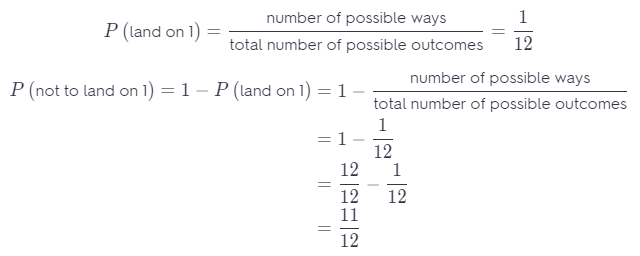The probability not to land on 1 is $$\frac{11}{12}$$.

Question 3.
Tania flips a coin three times. The coin lands on heads twice and on tails once, not necessarily in that order.
Number of possible ways is 3 because we can flip HHT, HTH, THH
Total number of possible outcomes is 8 because we can flip HHH, HTT, HHT, TTT, THH, TTH, THT, HTH.
P (lands on heads twice and on tails once) =The probability that coin lands on heads twice and on tails once is $$\frac{3}{8}$$.

Question 4.
Students are randomly assigned two-digit codes. Each digit is either 1, 2, 3, or 4. Guy is given the number 11.
Number of possible ways is 1 because to have 1 on each digit of code is only one.
Total number of possible outcomes is 16 because for first digit we have 4 possibilities and also for second so, 4 ∙ 4 = 16.
P (two-digit code is 11) =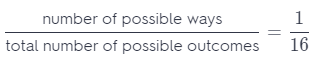The probability to give a number is 11 is $$\frac{1}{16}$$.

Question 5.
Patty tosses a coin and rolls a number cube. (Lesson 6.3)
a. Find the probability that the coin lands on heads and the cube lands on an even number.
Number of possible ways is 3 because there are three ways to flip a head and roll a even number: H-2,H-4, H-6.
Total number of possible outcomes is 12 because: for coin we have 2 possibilities and for cube 6, so 2 ∙ 6 = 12.
P (flip a head and a cube is on an even number) =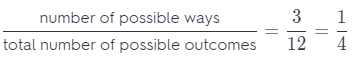The probability to flip a head and a cube is on an even number is $$\frac{1}{4}$$.

b. Patty tosses the coin and rolls the number cube 60 times. Predict how many times the coin will land on heads and the cube will land on an even number.
Use proportion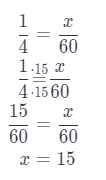The coin will land on head and a cube on an even number about 15 times out of 60.

Question 6.
Rajan’s school is having a raffle. The school sold raffle tickets with 3-digit numbers. Each digit is either 1, 2, or 3. The school also sold 2 tickets with the number 000. Which number is more likely to be picked, 123 or 000? (Lesson 6.3)
For total number of possible outcomes we have for first digit 3 possibilities, for second and third also and we have also 2 possibilities with 000, so 29 possibilities.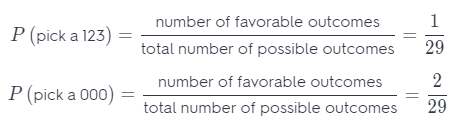It is more likely to pick a ticket numerated with 000 than ticket numerated with 123.

Question 7.
Suppose you know that over the last 10 years, the probability that your town would have at least one major storm was 40%. Describe a simulation that you could use to find the experimental probability that your town will have at least one major storm in at least 3 of the next 5 years. (Lesson 6.4)
The probability that the city wilt have at least one major storm is $$\frac{40}{100}$$ = $$\frac{2}{5}$$.
Let numbers 1 and 2 represent that there was a major storm, and 3 4 and 5 that not We generate five numbers in each of 10 trials Find the experimental probability that the major storm occurred at least three times of the five years.

Question 1.
CAREERS IN MATH Meteorologist A meteorologist predicts a 20% chance of rain for the next two nights, and a 75% chance of rain on the third night.
a. On which night is it most likely to rain? On that night, is it likely to rain or unlikely to rain?
P (chance to rain to the next two nights) = $$\frac{1}{5}$$
P (chance to rain third night) = $$\frac{3}{4}$$
Chance to rain is more likely third day than first two nights. It is likely to rain that night.

b. Tara would like to go camping for the next 3 nights, but will not go if it is likely to rain on all 3 nights. Should she go? Use probability to justify your answer.
P (chance to rain all three nights is) : $$\frac{1}{5}$$ ∙ $$\frac{3}{4}$$ = $$\frac{3}{20}$$
It is not likely to rain all three nights.

Question 2.
Sinead tossed 4 coins at the same time. She did this 50 times, and 6 of those times, all 4 coins showed the same result (heads or tails).
a. Find the experimental probability that all 4 coins show the same result when tossed.
The experimental probability that all 4 coins show the same result when tossed is: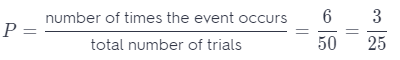b. Can you determine the experimental probability that no coin shows heads? Explain.
The experimental probability that all 4 coins shows heads is P = $$\frac{3}{25}$$.
The experimental probability that no coin shows the heads is:
1 – P = 1 – $$\frac{3}{25}$$ = $$\frac{25}{25}$$ – $$\frac{3}{25}$$ = $$\frac{22}{25}$$

c. Suppose Sinead tosses the coins 125 more times. Use experimental probability to predict the number of times that all 4 coins will show heads or tails. Show your work.
Use proportion.You can expect that all 4 coins will show heads or tails about 15 times out of 125.

Texas Go Math Grade 7 Unit 3 Mixed Review Texas Test Prep Answer Key

Selected Response

Question 1
A pizza parlor offers thin, thick, and traditional style pizza crusts. You can get pepperoni, beef, mushrooms, olives, or peppers for toppings. You order a one-topping pizza. How many outcomes are in the sample space?
(A) 3
(B) 5
(C) 8
(D) 15
(D) 15

Explanation:
3 possibilites for crust and 5 possibilities for topping are 3 ∙ 5 = 15 possibilities for pizza.
The sample space is 15.

Question 2.
A bag contains 9 purple marbles, 2 blue marbles, and 4 pink marbles, The probability of randomly drawing a blue marble is $$\frac{2}{15}$$. What is the probability of not drawing a blue marble?
(A) $$\frac{2}{15}$$
(B) $$\frac{4}{15}$$
(C) $$\frac{11}{15}$$
(D) $$\frac{13}{15}$$
(D) $$\frac{13}{15}$$

Explanation:The probability not to pick a blue marble is $$\frac{13}{15}$$.

Question 3.
During the month of April, Dora kept track of the bugs she saw in her garden. She saw a ladybug on 23 days of the month. What is the experimental probability that she will see a ladybug on May 1?
(A) $$\frac{1}{23}$$
(B) $$\frac{7}{30}$$
(C) $$\frac{1}{2}$$
(D) $$\frac{23}{30}$$
(D) $$\frac{23}{30}$$

Explanation:
Number of times the event occurs represents how many times a ladybug appeared in April.
Total number of trials represents how many days she kept track the bugs in her garden.
The experimental probability that she will see a ladybug on May 1 is:Question 4.
Ryan flips a coin 8 times and gets tails all 8 times. What is the experimental probability that Ryan will get heads the next time he flips the coin?
(A) 1
(B) $$\frac{1}{2}$$
(C) $$\frac{1}{8}$$
(D) 0
(D) 0

Explanation:
The experimental probability that Rayan flip a head next time is:
P (flip a head) =Question 5.
Jay tossed two coins several times and then recorded the results in the table below.What is the experimental probability that the coins will land on different sides on his next toss?
(A) $$\frac{1}{5}$$
(B) $$\frac{2}{5}$$
(C) $$\frac{3}{5}$$
(D) $$\frac{4}{5}$$
(C) $$\frac{3}{5}$$

Explanation:
Number of possible ways is 3 because in toss 1, 3, 5 we have that possibility.
P (the coins land on different sides) = $$\frac{3}{5}$$
The experimental probability that the coins land on different sides is $$\frac{3}{5}$$.

Question 6.
A used guitar is on sale for $280. Derek offers the seller $$\frac{3}{4}$$ of the advertised price. How much does Derek offer for the guitar? (A)$180
(B) $210 (C)$240
(D) $270 Answer: $$\frac{3}{5}$$ Explanation: Derek offer’s: 2800 ∙ $$\frac{3}{4}$$ = 210 Derek offer’s for the guitar$ 210.

Question 7.
What is the probability of tossing two fair coins and having exactly one land tails side up?
(A) $$\frac{1}{8}$$
(B) $$\frac{1}{4}$$
(C) $$\frac{1}{3}$$
(D) $$\frac{1}{2}$$
(D) $$\frac{1}{2}$$

Explanation:
Number of possible ways is 2 because we have HT or TH.
P (one land tails side up) =The probability of tossing two fair coins and having exactly one land tails side up is $$\frac{1}{2}$$.

Question 8.
Find the percent change from 60 to 96.
(A) 37.5% decrease
(B) 37.5% increase
(C) 60% decrease
(D) 60% increase
(D) 60% increase

Explanation
Use proportionSubtract 100 % of the last result. In this way, we will get a percentage of increase because we have taken away the original price from the increased price.
160 – 100 = 60%
The percent of change is 60% increase.

Question 9.
Jason, Erik, and Jamie are friends in art class. The teacher randomly chooses 2 of the 21 students in the class to work together on a project. What is the probability that two of these three friends will be chosen?
(A) $$\frac{1}{105}$$
(B) $$\frac{1}{70}$$
(C) $$\frac{34}{140}$$
(D) $$\frac{4}{50}$$
(B) $$\frac{1}{70}$$

Explanation:
Number of favorable possibilities:
3 for first student and 2 for second 6
Total number of possible outcomes:
21 for first student and 20 for second = 420.
P (two of three friends are chosen) =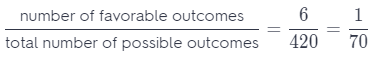The probability that two of these three students will be chosen is $$\frac{1}{70}$$.

Question 10.
Philip rolls a number cube 12 times. Which is the best prediction for the number of times that he will roll a number that is odd and less than 5?
(A) 2
(B) 3
(C) 4
(D) 6
(C) 4

Explanation: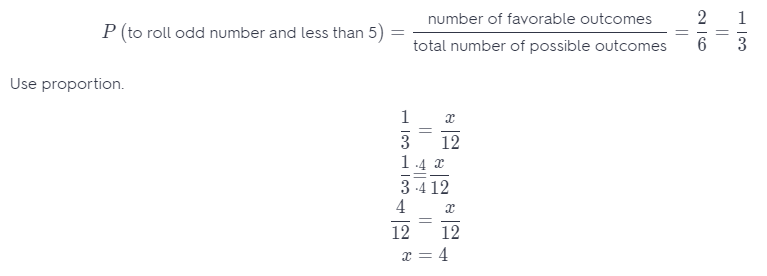Phillip can expect that he will roll a number that is odd and less than 5 about 4.

Gridded Response

Question 11.
A bag contains 6 white beads and 4 black beads. You pick out a bead at random, record its color, and put the bead back in the bag. You repeat this process 35 times. How many times would you expect to remove a white bead from the bag?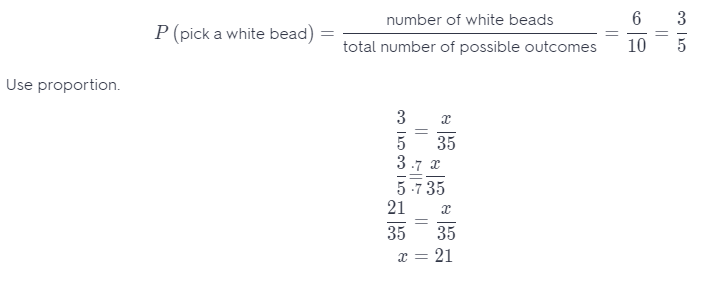How we get the value of 21 in the forth column from the left we round the number 2, in the column to the right of it 1. In the columns after the column with a decimal point we do not round anything because it is an integer.
You can expect that you will pick a white bead about 21 times

Question 12.
A set of cards includes 20 yellow cards, 16 green cards, and 24 blue cards. What is the probability, written in decimal form, that a blue card is chosen at random?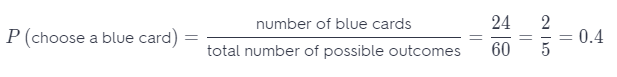As we get the decimal number 0.4 in the column in front of the column with a decimal point, we will round the number 0, while in the column after the decimal point we will round the number 4.
The probability to choose blue card is 0.4.

Question 13.
A survey reveals that one airline’s flights have a 92% probability of being on time. Out of 4,000 flights in a year, how many flights would you predict to arrive on time?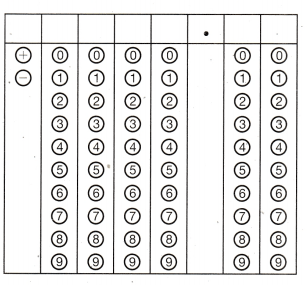P (flight arrive on time) = $$\frac{92}{100}$$ = $$\frac{23}{25}$$
To predict how many flights will arrive on time we use proportion. In that proportion equalize the probability that flight will arrive on time with fraction, which numerator is unknown and mean the number of flight which arrive on time out of 4000 flights, denominator 4000 which is total number of flights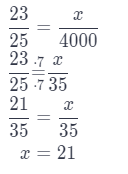Use the puzzle to preview key vocabulary from this unit. Unscramble the circled letters within found words to answer the riddle at the bottom of the page.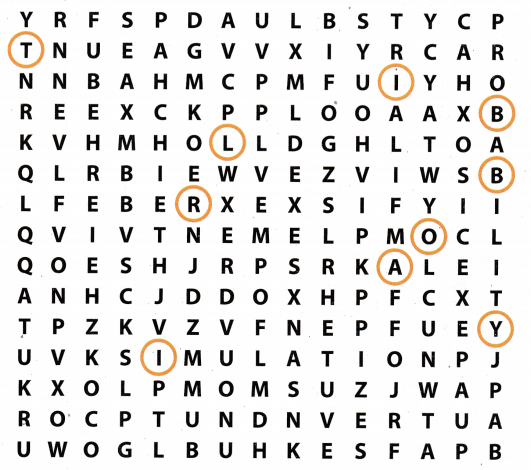• An activity based on chance in which results are observed. (Lesson 5-1)
• The set of all outcomes that are not included in the event. (Lesson 5-1)
• Each observation of an experiment. (Lesson 5-1)
• A model of an experiment that would be difficult or too time-consuming to perform. (Lesson 5-2) .
• Measures the likelihood that the event will occur. (Lesson 5-1)
• An event with only one outcome (2 words). (Lesson 5-2)
• A set of all possible outcomes for an event (2 words), (Lesson 5-1)

Question.
Why was there little chance of success for the clumsy thieves?